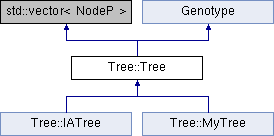# Tree::Tree Class Reference [Genotypes, Tree]

Tree class - implements genotype as a tree. More...

`#include <Tree_c.h>`

Inheritance diagram for Tree::Tree:List of all members.

## Public Member Functions

Treecopy ()
Create an identical copy of the genotype object.
void growBuild (PrimitiveSetP primitiveSet)
Build tree with grow method.
void fullBuild (PrimitiveSetP primitiveSet)
Build tree with full method.
void update ()
Calculate depth and subtree sizes of each node in the tree.
void execute (void *)
Execute current tree.
void addNode (Node *node)
void addNode (NodeP node)
void setTerminalValue (std::string, void *)
Set a terminal's value.
void getTerminalValue (std::string, void *)
Retrieve a terminal's value.
void write (XMLNode &)
Write genotype data to XMLNode.
void read (XMLNode &)
Read genotype data from XMLNode.
void registerParameters (StateP)
Register genotype's parameters (called before Genotype::initialize).
bool initialize (StateP state)
Initialize a genotype object (read parameters, perform sanity check, build data).
std::vector< MutationOpP > getMutationOp ()
Create and return a vector of mutation operators.
std::vector< CrossoverOpP > getCrossoverOp ()
Create and return a vector of crossover operators.
bool addFunction (PrimitiveP)
Add user defined function primitive. Must be called prior to initialization (no impact otherwise).
bool addTerminal (PrimitiveP)
Add user defined terminal primitive. Must be called prior to initialization (no impact otherwise).
uint getMaxDepth ()
uint getMinDepth ()
uint getStartDepth ()

## Public Attributes

PrimitiveSetP primitiveSet_
uint iNode_
current node index (when parsing the tree)
uint startDepth_
start depth of the first node (0 by default)
uint maxDepth_
max allowed Tree depth
uint minDepth_
min allowed Tree depth
uint initMaxDepth_
max allowed Tree depth at initialization (maxDepth_ used if not defined)
uint initMinDepth_
min allowed Tree depth at initialization (minDepth_ used if not defined)

## Protected Member Functions

uint growBuild (PrimitiveSetP primitiveSet, int myDepth)
Build Tree using 'grow' method.
uint fullBuild (PrimitiveSetP primitiveSet, int myDepth)
Build Tree using 'full' method.
uint setSize (int)
Calculate subtree sizes of each node in the tree.
void setDepth (int myDepth)
Calculate depth of each node in the tree.
virtual void initializeFirst (Tree *hometree)
Performs the first Tree initialization (performed only once for each active Tree Genotype). Constructs the primitive set and performs parameter checks.

## Protected Attributes

std::vector< PrimitiveP > userFunctions_
std::vector< PrimitiveP > userTerminals_
StateP state_

## Detailed Description

Tree class - implements genotype as a tree.

Tree inherits a vector of Node objects. Each node points to a Primitive object. Nodes in tree are aligned in a prefix notation. Some primitives are shared among all the trees, but some (such as ERC primitives) belong to a single Tree.

Definition at line 28 of file Tree_c.h.

## Member Function Documentation

 void Tree::Tree::execute ( void * result )

Execute current tree.

Evaluates the function embodied in the tree (or executes the represented program).

Parameters:
 result the resulting tree value (of the first node)

Definition at line 362 of file Tree.cpp.

 void Tree::Tree::getTerminalValue ( std::string name, void * value )

Retrieve a terminal's value.

Parameters:
 name terminal's name value terminal's value

Definition at line 522 of file Tree.cpp.

 void Tree::Tree::initializeFirst ( Tree * hometree ) ` [protected, virtual]`

Performs the first Tree initialization (performed only once for each active Tree Genotype). Constructs the primitive set and performs parameter checks.

Parameters:
 hometree uninitialized Tree instance in vector of active Genotypes stored in State

Definition at line 149 of file Tree.cpp.

 void Tree::Tree::setTerminalValue ( std::string name, void * value )

Set a terminal's value.

Parameters:
 name terminal's name value terminal's value

Definition at line 504 of file Tree.cpp.

 void Tree::Tree::update ( void )

Calculate depth and subtree sizes of each node in the tree.

Uses recursive depth-first tree processing.

Definition at line 435 of file Tree.cpp.

The documentation for this class was generated from the following files: